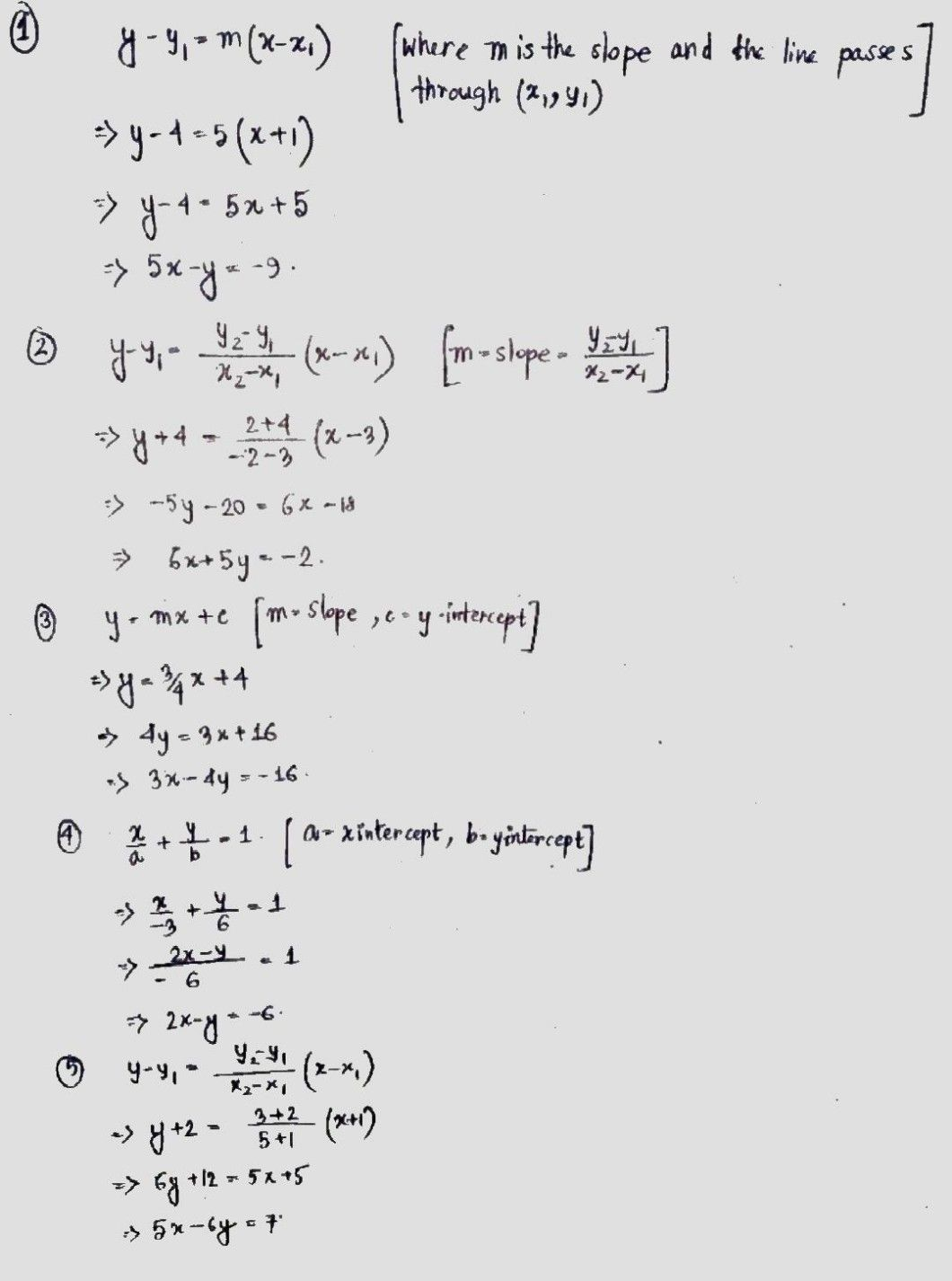Symbol
ProblemLearning Task 3. Find the equation of the line. Do it in your notebook. (a) In slope intercept form $y=mx+b$ $1b1$ in standard form $ax+by=c$ $1$ The slope $s5$ passing through $1-1,4\right)$ $2$ The line passes through point $13$ -4) $and$ $\left(-2,2\right)$ $y-lntcrccp$ $ls\left(0.4\right)$ $3$ The slope is 3 and the 4. The x intercept -3 and the $y-intcrccp$ $106$ $5$ Passing through the points $1=1$ -2) and $15,3\right)$
7th-9th grade
Other
Search count: 107
SolutionQanda teacher - AskShivaniIf you have any doubt feel free to ask:) Dear, Please evaluate the answer and give gift coins(it does not reduce your coin balance.) for giving gift coins Click on evaluate. Then press the + icon 3 times(3000).
Dear, Please evaluate the answer and give gift coins(it does not reduce your coin balance.) for giving gift coins Click on evaluate. Then press the + icon 3 times(3000).Student
thank youQanda teacher - AskShivani
welcome dear
Dear, Please evaluate the answer and give gift coins(it does not reduce your coin balance.) for giving gift coins Click on evaluate. Then press the + icon 3 times(3000).